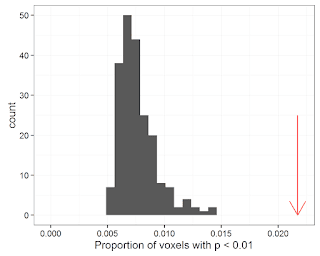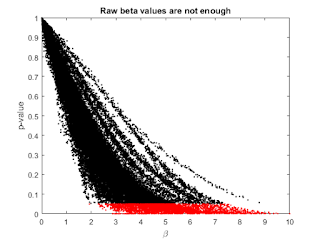## Monday, April 16, 2018

### Correcting for multiple comparisons in lesion-symptom mapping

We recently wrote a paper about correcting for multiple comparisons in voxel-based lesion-symptom mapping (Mirman et al., in press). Two methods did not perform very well: (1) setting a minimum cluster size based on permutations produced too much spillover beyond the true region, (2) false discovery rate (FDR) correction produced anti-conservative results for smaller sample sizes (N = 30–60). We developed an alternative solution by generalizing the standard permutation-based family-wise error correction approach, which provides a principled way to balance false positives and false negatives.

For that paper, we focused on standard "mass univariate" VLSM where the multiple comparisons are a clear problem. The multiple comparisons problem plays out differently in multivariate lesion-symptom mapping methods such as support vector regression LSM (SVR-LSM; Zhang et al., 2014, a slightly updated version is available from our github repo). Multivariate LSM methods consider all voxels simultaneously and there is not a simple relationship between voxel-level test statistics and p-values. In SVR-LSM, the voxel-level statistic is a SVR beta value and the p-values for those betas are calculated by permutation. I've been trying to work out how to deal with multiple comparisons in SVR-LSM.

My first (in retrospect, irrationally optimistic) idea, was that, since this is a multivariate analysis method that considers all voxels simultaneously, they do not constitute multiple comparisons and therefore no correction is necessary. I was running permutations to get the p-values, so I tweaked the code to record all of the beta values for all of the permutations, which allowed me to calculate p-values for the original (true) analysis as well the permuted (null) analyses. In the permutation analyses, where there was (by definition) no relationship between lesion location and behavioral deficit score, the p-values were literally true: with the null hypothesis being true, the proportion of voxels with p-values less than some level alpha was equal to alpha. The histogram below shows the distribution of proportion of voxels with (permutation-based) p < 0.01 in 210 permutations. In general, about 0.5-1% of the voxels had (uncorrected) p < 0.01, as is expected under the null hypothesis.When you're testing a very large number of voxels, this is a big problem: when 100,000 voxels are in play, even if the null hypothesis is true, about 500-1000 voxels are going to have (uncorrected) p < 0.01. The red arrow shows that proportion for the true analysis (in which there really was a relationship between lesion location and deficit score), which was 0.0217 (2.17%). It is encouraging that the true analysis had substantially more voxels with p < 0.01, but it would still be hard to interpret a result when nearly half of it could be due to chance.

So some kind of correction would be helpful. Since the p-values are based on permutation, using more permutations to correct for multiple comparisons (that is, a standard permutation-based FWER correction or our generalized version) would be redundant. My second idea was that we could calculate a correction for the SVR beta values in the same way that standard FWER correction works on voxel-level test statistics. But the SVR beta values are not test statistics in the same way that voxel-level t-values are test statistics. Specifically, there is not a unique, one-to-one relationship between beta value and p-value. The figure below shows a scatterplot of voxel-wise beta values (x-axis) and p-values (y-axis). The p<0.05 points are shown in red.In general, larger beta values tend to have smaller p-values, which makes good sense, but there is a huge amount of variability. Some relatively low beta values (3-4) have very low p-values while others have very high p-values; most relatively high beta values (7-9) have low p-values, but some don't. I suspect that locations near the periphery of the lesion territory are more prone to large beta values because fewer patients have damage there, so large beta values there are less meaningful than in other locations, but I haven't checked that. Bottom line: a multiple comparisons correction can't be based on raw beta values.

So what options are left?

(1) Cluster-based correction. One could set a voxel-wise p-value (e.g., p < 0.01) and use permutations to determine a null distribution of maximal cluster sizes for that p-value, then only consider clusters that are larger than (say) 95% of the null distribution of clusters. We tested this for mass univariate LSM and found that it produces clusters that tend to spill far beyond the true regions. Using this method for SVR-LSM would be better than doing nothing, but I think it would have the same problem as we identified for mass univariate LSM. Also, since multivariate LSM is especially good for detecting separately contributing regions, focusing on particularly large clusters would undermine that advantage of the method.

(2) False Discovery Rate (FDR). For mass univariate LSM, we found that FDR tended to be somewhat anti-conservative for smaller samples and real data (it performed reasonably well for larger samples and simulated data, where the lesion-symptom relationship is very strong). It's not clear to me whether this would also apply to SVR-LSM, but even if it does, it would still be better than no correction. At FDR < 0.05, we expect that up to 5% of above-threshold voxels may be false positives. Even if that is an anti-conservative estimate and up to 10% (or even 15%) of the above-threshold voxels might be false positives, that is still a relatively small subset of the voxels, which probably won't affect my inference.

FDR is widely used for multiple comparisons correction and relatively easy to implement, so I think the best strategy at this point is to use FDR, but be aware that it might be somewhat anti-conservative, and to supplement it with some kind of minimum cluster threshold to avoid interpreting small clusters that could easily arise by chance.

References

1. Mirman, D., Landrigan, J.-F., Kokolis, S., Verillo, S., Ferrara, C., & Pustina, D. (2018). Corrections for multiple comparisons in voxel-based lesion-symptom mapping Neuropsychologia. DOI: 10.1016/j.neuropsychologia.2017.08.025
2. Zhang, Y., Kimberg, D. Y., Coslett, H. B., Schwartz, M. F., & Wang, Z. (2014). Multivariate lesion-symptom mapping using support vector regression Human Brain Mapping, 35 (12), 5861-5876. DOI: 10.1002/hbm.22590